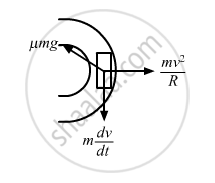Share

# A Car Goes on a Horizontal Circular Road of Radius R, the Speed Increasing at a Constant Rate D V D T = a . the Friction Coefficient Between the Road and the Tyre is μ. - Physics

ConceptCircular Motion

#### Question

A car goes on a horizontal circular road of radius R, the speed increasing at a constant rate $\frac{\text{dv}}{\text{dt}} = a$ . The friction coefficient between the road and the tyre is μ. Find the speed at which the car will skid.

#### Solution

Let v be the speed of the car.
Since the motion is non-uniform, the acceleration has both radial (ar) and tangential (at) components.

$\text{a}_\text{r} = \frac{\text{v}^2}{\text{R}}$

$\text{a}_\text{t} = \frac{\text{dv}}{\text{dt}} = a$

$\text { Resultant magnitude } = \sqrt{\left( \frac{\text{v}^2}{R} \right)^2 + a^2}$From free body diagram, we have :

$\text{mN = m}\sqrt{\left( \frac{\text{v}^2}{R} \right)^2 + a^2}$

$\Rightarrow \mu \text{ mg = m}\sqrt{\left( \frac{\text{v}^2}{R} \right)^2 + a^2}$

$\Rightarrow \mu^2 g^2 = \frac{\text{v}^4}{R^2} + a^2$

$\Rightarrow \frac{\text{v}^4}{R^2} = ( \mu^2 g^2 - a^2 )$

$\Rightarrow \text{v}^4 = ( \mu^2 g^2 - a^2 ) R^2$

$\Rightarrow \text{v} = [( \mu^2 g^2 - a^2 ) R^2 ]^{1/4}$

Is there an error in this question or solution?

#### APPEARS IN

Solution A Car Goes on a Horizontal Circular Road of Radius R, the Speed Increasing at a Constant Rate D V D T = a . the Friction Coefficient Between the Road and the Tyre is μ. Concept: Circular Motion.
S